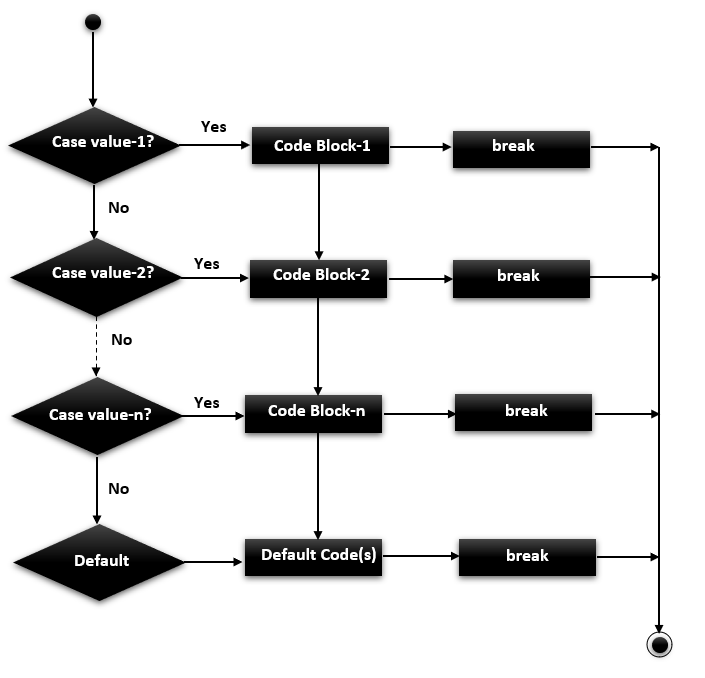# C++ Switch Case Statement

In this tutorial, you will learn how to create the switch statement in the C++ programming language with the help of an example.

In C++, If you want to make a type of decision where you will be matching the value of a variable with several constant or integer values, you can use a switch case statement. It is similar to `if...else...if` ladder statement but the only difference is that it checks for equality of the value provided in the switch statement with the various cases provided. One thing to remember that all the cases need to be unique. If none of the cases match the value, you can provide a default case that will work like the else condition block and will get printed in that case.

## C++ switch case syntax

The syntax of the switch case in C++ is given below.

```switch(expression){
case value1:
//code to be executed;
break; //Break statement is necessary for all cases or else all
//consecutive cases will also get executed after matching
case value2:
//code to be executed;
break;
......
default:
code to be executed if all cases are not matched;
//Here break is not necessary as it is the last case.
}```

## Flowchart of switch case in C++## Rules of switch case in c++

In a program, you need to remember a few rules and features of the switch case to use them effectively which are as below.

• You cannot provide a Boolean value in the switch case and it needs to be get evaluated to a constant value for the variable provided in the switch case.
• The value must be either an integer, character, short or long type value. Other types of values will give an error.
• Duplicate cases with the same values will give an error.
• The default statement is optional and not necessary.
• The break keyword is also optional however it is required to avoid the execution of the following case statements after one case statement has been successfully matched.
• Nesting of switch case is possible by providing one switch case statement inside another but it makes the program very complicated and should be avoided if possible.

## C++ Switch Case Example

### Example 1: Switch Case with the break statement

Following is a simple C++ program to demonstrate the switch case with the break.

```#include <iostream>
using namespace std;
int main() {
int x = 2;
switch (x)
{
case 1:
cout << "Choice is 1";
break;
case 2:
cout << "Choice is 2";
break;
case 3:
cout << "Choice is 3";
break;
default:
cout << "Choice other than 1, 2 and 3";
break;
}
return 0;
}```

Choice is 2

### Example 2: Switch Case without break statement

Following is a simple C++ program to demonstrate the switch case without the break.

```#include <iostream>
using namespace std;
int main() {
int x = 2;
switch (x)
{
case 1:
cout << "Choice is 1";
case 2:
cout << "Choice is 2";
case 3:
cout << "Choice is 3";
default:
cout << "Choice other than 1, 2 and 3";
}
return 0;
}```

### Output

Choice is 2
Choice is 3
Choice other than 1,2 and 3

### Example 3: Switch Case without default statement

Following is a simple C++ program to demonstrate the switch case without a default statement.

```#include <iostream>
using namespace std;
int main() {
int x = 2;
switch (x)
{
case 1:
cout << "Choice is 1";
case 2:
cout << "Choice is 2";
case 3:
cout << "Choice is 3";   // If no cases match, nothing is printed
}
return 0;
}```

Choice is 2